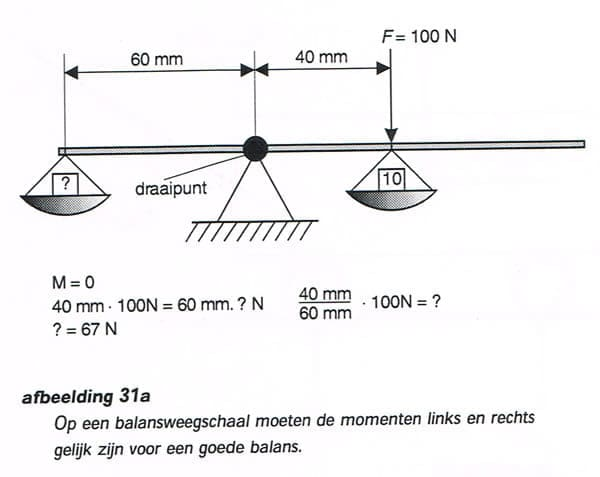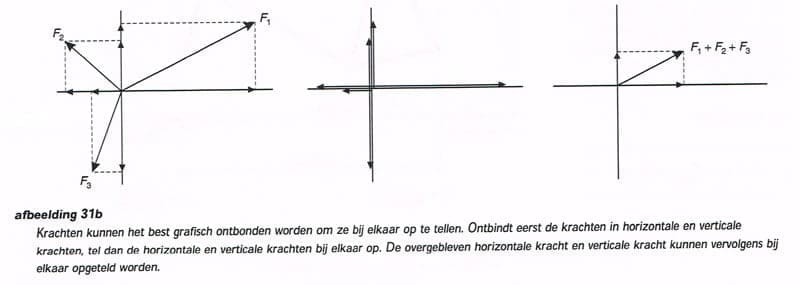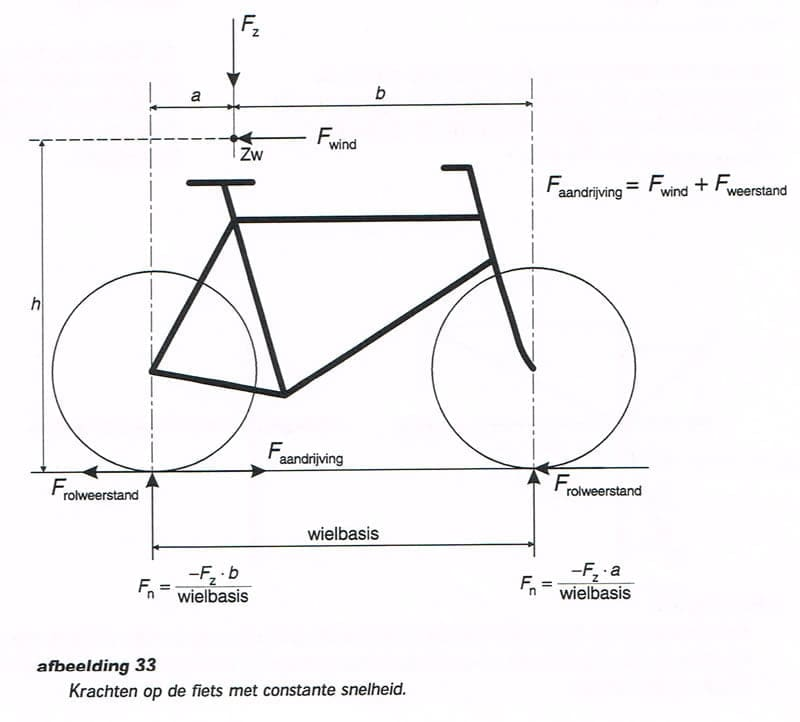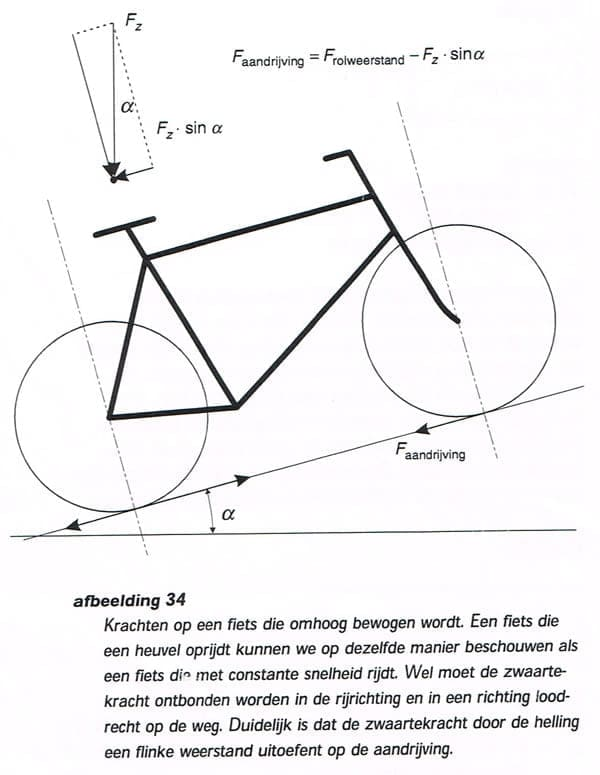General mechanical laws

General mechanical laws.

(The images on this page are redirected from the dutch pages and not yet translated)

On a bicycle energy from the treading force of the cyclist is converted into a forward movement. To understand the functioning of the various components in the bicycle it is useful to know some rules of mechanics and understand them. They are really not that hard.

The most important concept in mechanics is the Force.

If a force is aplied to an object
these three effects may result in :

• whether the object is accelerating
• or object remains still lying or standing
• or whether the object is moving with constant velocity..

If an object is not accelerating the environment should exert a reaction force on the object.

For objects which have not been at rest the following applies:

F = m x a

whereby:

F = the force in N
m = the mass in kg
a = the acceleration in m/s

On an object which falls down freely works only the force of gravity. As a result, the object will receive an acceleration and ever move faster. If there is a reaction force that acts over the reaction, force has a value of -F. The law that applies here is: action is reaction.

F action = F reaction

On an object lying on the ground operates the force of gravity. The object remains silent because the ground also exerts a force -F worth gravity.  See Img. 30.

A second important concept in mechanics is the Moment. If, at a distance of an object a force is exerted, which force exerts a moment on the object to the value of the force times the distance. Usually this distance is called arm or lever.

The formula is:

M = F x 1

whereby:

M = the moment in Nm
F = the force in N
1 = the distance in m.

For moments, the same applies as for forces. By moments an object can not move by itself, but it rotates on its axis. If a moment is exerted on an object it can start to rotate accelerated, it remains stationary, or rotates at a constant speed. If it stands still there has to be a reaction time toe that object. The reaction time has the same magnitude but opposite direction of rotation. With a balance scale it is good to see that the moments must be in balance.

If of 10 kg to the left of the of the balance point of rotation is placed a mass at a distance of 10 cm, and you place to the right of the pivot point a mass of 5 kg at a distance of 20 cm then the balance is in ‘balance’.

whereby:

M action = M reaction

Forces can be resolved into horizontal forces and vertical forces. This is useful because it can be looked whether there is horizontal and / or vertical balance. The easiest way to dissolve forces is by graphic. Forces that have the same direction and facing the same way may be added together, they are displayed graphically than two times as long. Forces that have the same direction but the opposite side may signal to be subtracted from each other. If the sum of the forces is 0 an object is at rest or continue to move at a constant speed. If the sum of the forces is not 0 it will start to move the object in the direction where the force is pointing to.

The following applies to the value of the force:

whereby:

(?) = the angle that the force will take withthe horizontal line.

For example, where a trolley is pulled by a rope will be given an acceleration in the horizontal direction. See Img. 32.

The value is:

m x a = F hand x cos (?)

In the vertical direction there is balance. The reaction force of the ground on the trolley is:

F reaction = F hand X Sin (?) – F gravityAn object in which a force or moment is exerted on will move at constant speed or remain stationary if the forces in the horizontal and vertical direction are equal and if the moments are equal. See Img. 31. Generally, this is formulated as follows: the sum of the forces and moments on an object at rest is equal to zero.

With this data , for example, the forces acting on a bicycle can be determined. If a bicycle runs at a constant speed, the following forces that act on: the force of gravity, the resistance due to the wind, the drive force to the rear wheel, the normal forces on the wheels, and the rolling resistance of the wheels (a force that is counteracting a movement resistance called). See Img. 33. If the rolling resistance, driving wind, place of the mass and center of gravity are known, the driving force and the reaction force can be calculated.The forces in the horizontal direction are in balance. This is F rear wheel = F driving wind + F rolling resistance

The forces in the vertical direction are in balance. This will make F rear wheel + F front wheel = F gravityUnfortunately, the reaction force on the front and rear wheels is not still to be evaluated. For this, also the sum of the moments are equal. The turning point where we calculate the moments must be chosen smart. Because the forces at the rear and front both are still unknown, it is convenient to calculate the moments of the front or rear wheels.

If we calculate it to the rear wheel, the equation is:

F front wheel x wheelbase + F driving wind x h – F gravity x a = 0

Usually the wind pressure is negligible, so that the reaction force on the front wheel –Fz x a/wheelbase and on the rear wheel –Fz x b/wheelbase.

With a bike it is not just about forces and moments. It also involves relocation. To move a force over a distance there has to be labor.When is pushed against an object without getting the object in motion, there’s labor. The labor force x distance traveled. It remains suspended, there is no distance traveled, so is force times path is zero.

Whereby:

W = F x s
W = labor in Nm
F = force in N
S = distance in m

The bike is to be regarded as a mechanical converter or amplifier. See Fig. 35. A mechanical transducer is a mechanism which converts an input force and displacement in an output force and displacement and the direction of the force can change. For example, the front chain wheel gets the vertical leg-power on the crank to be in a horizontal tensile force in the chain. The work that goes into a mechanical amplifier must also come out again.

F in x l in = F out x l out
F in = the force that is placed in the amplifier in N
l in = the displacement of the force in the amplifier in m
F uit = the force that comes out of the amplifier in N
l uit = the displacement from the amplifier in m.

The bicycle consists of a large number of mechanical transducers. See Img. 36.

The wheel, chain, sprockets, brake lever, cables and gear hub are all regarded as mechanical transducers. In studying the various components on the bike it is useful to see what is happening with the forces and movements and where possible losses are.Deze site gebruikt Akismet om spam te verminderen. Bekijk hoe je reactie-gegevens worden verwerkt.